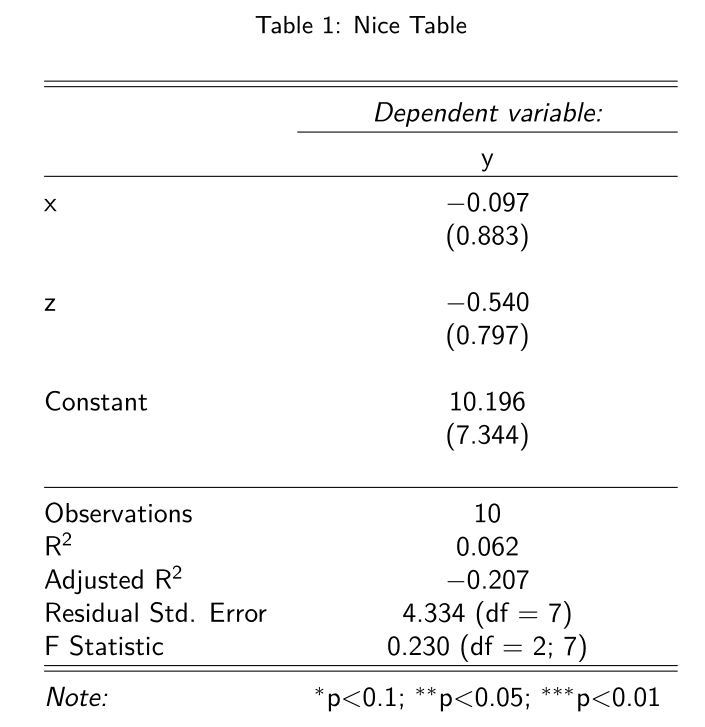## 9.2 Knitr: Rmarkdown

Rmarkdown is slightly more complicated to produce but the code is simpler. It is based on pandoc.

First, we create .Rmd RMarkdown file. Go to File > New File > RMarkdown

Then try to type something and then click the knit icon.

There are two ways to incorporate R code:

1. Inline: start with back ticks and r, end with back ticks

For example, 32+42 is equal to 25

1. Display: start with three back ticks and {r}, end with three backticks
3^2+4^2

This gives the following:

3^2+4^2
##  25

Since the Rmarkdown is based on pandoc, the syntax is also very similar:

This is plain text

This is *italics*. This is also _italitcs_

This is **bold**. This is also __bold__

To write a^2^, we need a^2^ 

For sectioning, we have

• Header 1: #
• Header 2: ##
• Header 3: ###

For list, we have unordered list and ordered list.

An unordered list is start with -. Add four spaces for a sub-list.

- I am a boy.
- You are a girl.
- He has an apple.

The result is:

• I am a boy.
• You are a girl.
• He has an apple.

For unordered list, the syntax is as follows:

1. I am a boy.
2. You are a girl.
1. He has an apple.

The result is:

1. I am a boy.
2. You are a girl.
1. He has an apple.

Mathematics is similar to the latex use dollar sign for inline equations:

$a^2+b^2=c^2$

$$a^2+b^2=c^2$$

Use double dollar sign for displayed equations:

$$a^2+b^2=c^2$$

$E = mc^2$ Below shows an application example:

getSymbols("AAPL")
tail(Cl(AAPL),5)
suppressMessages(library(stargazer))
x <- sample(1:5,10,TRUE)
z <- sample(5:10,10,TRUE)
y <- sample(1:10,10,TRUE)
df <- data.frame(x,y,z)
reg <- lm(y ~ x + z,data=df)
stargazer(reg,header = FALSE)

The above code will give: